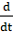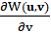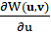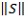# МОДЕЛИРОВАНИЕ ЭНЕРГЕТИЧЕСКОГО ГОМЕОСТАЗА В ПРОСТРАНСТВЕ ПЕРЕМЕННЫХ СОСТОЯНИЯ

Сучилин Владимир Александрович
Transoffice-Information GbR
Фильдерштадт (Германия), Технический директор

Аннотация
В статье рассматривается модель энергетического гомеостаза, в основу которой положен принцип наименьшего действия. Из этой модели следует, что при малых возмущениях механизм гомеостаза ведет себя подобно структурному звену динамической системы апериодического или колебательного типа. Исходя из этой аналогии, предложен подход к оценке устойчивости энергетического гомеостаза. В общем случае эта модель является нелинейной и при больших возмущениях приводит к предельным циклам и бифуркациям.

# STATE-SPACE MODELING OF THE ENERGY HOMEOSTASIS

Transoffice-Information GbR

Abstract
The article discusses a model of energy homeostasis, which only is based on the principle of least action. This model shows that by small disturbing influences, the homeostasis behaves like structural units of dynamic system of oscillation or aperiodic type. Based on the analogy, an approach to assessing the stability of energy homeostasis is proposed. In general this model is nonlinear and by more significant disturbing influences leads to limit cycles or bifurcation points.

Библиографическая ссылка на статью:
Сучилин В.А. State-Space Modeling of the Energy Homeostasis // Современные научные исследования и инновации. 2019. № 11 [Электронный ресурс]. URL: https://web.snauka.ru/issues/2019/11/90475 (дата обращения: 03.12.2023).

Introduction

In a broad sense, energy homeostasis ensures the maintenance of the dynamic balance of the system under disturbing influences, for example, in connection with changes in external conditions . Dynamic models of homeostasis at the level of individual bio-systems were considered in  – . It has also been suggested that some extreme principle is at the core of homeostasis at any level. . In physics, this one is the universal principle of least action . It is shown below that basing only on this principle it is possible to formulate a mathematical state-space model of energy homeostasis. Therefore, we call it as the principle model of energy homeostasis.

The principle model’s idea of energy homeostasis

In the most general formulation, the principle of least action reduces to the fact that the behavior of the system in question should provide a minimum of the Hamilton functional. Further, the use of this principle leads to the second-order Lagrange equation :–= 0       (1)

where:
u and v - vectors whose components are state-space variables qi(t) and q̇i(t)
W(u;v) – energy function

We will proceed from the fact that the function of the energy state can be represented as a positive definite quadratic form:

W(u;v) = 0.5 YSY>0       (2)

where:
Y - vector of state-space variables
S – real symmetric matrix whose elements are determined by the parameters of
homeostasis.
So in case of two variables Y = {q(t),q̇(t)} and:

S =(3)

Substituting (3) into (2), we obtain the explicit expression for the energy state function:

W(q(t),q̇(t)) = 0.5 (a∙q(t)2+2c∙q(t)∙q̇(t)+b∙q̇(t)2)        (4)

Further, substitution of (4) in (1) leads to differential equation:

h∙q̈(t) + g∙q̇(t) = 0     (5)

where: h = b – c and g = c – a.

For a nontrivial solution of this equation, disturbing influences function F(q(t),q̇(t)) should be introduced into the right-hand side of (5). Then the ordinary equation (5) takes the form:

h∙q̈(t) + g∙q̇(t) = F(q(t),q̇(t))       (6)

Note, equation (6) is a generalization of the models formulated for some specific bio-systems -.

Here, to make clear some possible solutions we consider two main particular cases of the principle model of energy homeostasis depending on the specific representation of the function F(q(t),q̇(t)).

Linear case

In order to represent the function F (q (t), q̇ (t)) in closed form in case of small disturbing influences, we restrict ourselves to the first terms of the Taylor series expansion near the balance point of homeostasis. So in this linear case, omitting the intermediate calculations, we obtain:

F(q(t),q̇(t)) = α+ β∙q(t) + γ∙q̇(t)          (7)

where: бв and г – coefficients of Taylor series.

Considering equation (5), it leads to differential equation of the second order

h∙q̈(t) + (g-γ) q̇(t) – β∙q(t) – α = 0          (8)

Using the substitution s(t) = – (б+в·q(t)), finally obtain:

ā∙s̈(t) + c̄∙ṡ(t) + s(t) = 0            (9)

where: aЇ = –h/в and cЇ = (г – g)/в.

In particular aЇ = 0, and equation (9) reduces to the differential equation of the first order:

c̄∙ṡ(t) + s(t) = 0        (10)

Note, analogs of equations (9) and (10) are, respectively, the oscillation and aperiodic structural units, which correspond to the cybernetic interpretation of homeostasis as a dynamic system with feedback .

Non-linear case

In general, the function on the right-hand side of equation (1) can be represented with Taylor series with terms of the second and higher orders or with a specific non-linear expression. As example of the latter, consider the disturbing influences function:

F(q(t),q̇(t)) = g∙q̇(t)q(t)2 – q(t)      (11)

Then, using (11) in the right-hand side of equation (5) we obtain:

h∙q̈(t) + g∙(1-q(t)2)∙q̇(t) + q(t) = 0       (12)

Actually, this equation describes the well-known Van der Pol oscillator, which is frequently used as a model of the processes in electrical or biological systems .

This oscillator is characterized by limit cycles and bifurcation points. However, its oscillations are harmonic at g = 0, and with an increase of |g| deviate more and more from harmonic oscillations approaching ultimately the relaxation oscillations. In addition, in the light of dependence of this nonlinear model on the choice of an inertial reference frame, it points to a new, relativistic aspect of homeostasis study .

Discussion

A possible problem of the presented principle model of energy homeostasis is that the state variables here are not directly related to the actual parameters of homeostasis. Therefore, the practical use of this model should be preceded by an assessment of its parameters. Here we confine ourselves to pointing to methods of identification theory that can be used for such an assessment .

As shown above, by small disturbing influences, the principle model of energy homeostasis is similar to linear dynamic systems. Therefore, to assess the stability of the energy homeostasis, one can use the known criteria of dynamic systems . In a more general case, the principle model is nonlinear and, with the more significant disturbing influences, leads to limit cycles. Thus, it allows one to study energy homeostasis coincided with external influences of a cyclic type .

Conclusions

Above the principle model of energy homeostasis, which only is based on the principle of least action was considered. This model shows that by small disturbing influences, the homeostasis behaves like structural units of dynamic system of oscillation or aperiodic type. Based on the analogy, an approach to assessing the stability of energy homeostasis is proposed. In general this model is nonlinear and by more significant disturbing influences shows the limit cycles or bifurcation points. In addition, in the light of dependence of this nonlinear model on the choice of an inertial reference frame, it points to a new, relativistic aspect of homeostasis study.

References
1. Wiener N. Cybernetics or Control and Communication in the Animal and the Machine. John Wiley & Sons Inc.: New York, 1948, 212 p.
2. Lombarte M. et al. Mathematical model of glucose-insulin homeostasis in healthy rats. Math Biosci. Oct 2013, 245(2), p. 269-277.
3. Kobayashi Y. et al. Mathematical model for calcium-assisted epidermal homeostasis. Journal of Theoretical Biology. May 2016 5(397), p. 52-60
4. Хлебович Н. Н. Уровни гомеостаза. Refbank #15536 [Электронный ресурс]. URL: https://www.docsity.com/ru/urovni-gomeostaza/1364816/
5. Полак Л. С. Вариационные принципы механики. M: Физматлит. 1959, 932 с.
6. Неймарк Ю. И. Динамические системы и управляемые процессы. М: Наука, 1978, 336 с.
7. Gatland I. R. Theory of a nonlinear oscillator. Am. J. Phys. 59, 1991, p. 155-158
8. Soutchilin V. Relativistic Time Dilation and Stability of Nonlinear Dynamical Systems // Современные научные исследования и инновации. 2018. № 3 [Электронный ресурс]. URL: http://web.snauka.ru/issues/2018/03/86003
9. Wang L., Garnier H. System Identification, Environmental Modelling, and Control System Design. Springer Science & Business Media: 2011, 648 p.